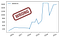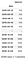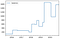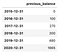# Handling Monetary Ledgers with Pandas, Python

A ledger is a table that records monetary trasactions, as credit or debit, and is always indexed in a chronological way, by date and time. It is used since ancient times to log account transactions and tell and reconstruct the history of events on that account.

Ledger is a fundamental data management tool implemented in systems of banks and financial institutions. Before computer systems, banks already used ledgers as regular paper books to manage their clients accounts.

Here is an example of a ledger recording transactions that happened on an account across 5 years. Positive transaction values are credits to that account, while negative values are debits or withdrawals.

Let’s use code to create our exemplary ledger:

`import pandas as pdledger=pd.DataFrame(    columns=['date'  ,  'value', 'comment'],    data=[        ('2015-05-15',   100   , 'Salary'),        ('2016-02-06',   200   , 'Project income'),        ('2016-08-10',   -30   , 'Gift for Max'),        ('2017-10-14',   -50   , 'Fix car'),        ('2018-02-01',   400   , 'Project income'),        ('2018-06-06',   -20   , 'Lunch with family'),        ('2018-08-09',   200   , 'Project income'),        ('2018-11-02',  -300   , 'House mortgage'),        ('2019-03-22',   200   , 'Salary'),        ('2019-05-18',  1000   , 'Ambitious project income'),        ('2019-12-24',   -15   , 'Christmas gift for Clara'),        ('2020-02-10',  -700   , 'House final mortgage'),        ('2020-08-01',   600   , 'Project income'),        ('2020-12-09',    20   , 'Last part of project income')    ])# Convert the textual `date` column into true datetimeledger.date=pd.to_datetime(ledger.date)# Make it the index of our dataframeledger.set_index('date', inplace=True)`

Although they might look like ones, ledgers are not what we use to call time series. A ledger holds a lot of semantic but we’ll have to convert it into a time series in order to make it useful in calulations and plots or even predictions. The ledger seen as a time series is a table with the total balance at that point in time. For that we’ll use Panda’s `cumsum()` function that computes the cumulative sum — also known as balance — at each data point.

`ledger_as_timeseries = ledger[['value']].cumsum()`

Don’t forget to put in chronological order before making calculations with `cumsum()`, otherwise we'll get completely meaningless results. Our example is already sorted, but just in case:

`balance = (    ledger[['value']]    .sort_index()    .cumsum()    .rename(columns={'value': 'balance'}))`

This table is now a genuine time series. It conveys the ups and down of the balance across those 5 years of account history. It says that after its inception in 2015, with a credit of 100, it received more values but had withdrawals too, had a big credit in May 2019 and finished 2020 with a balance of 1605. Can we plot it? Let’s try (even if we are not ready yet).

`balance.plot()`First attempt to plot the balance as a ragged time series

This plot is wrong. Balance in 2015 did not grow continuosly as the line shows. Ledger data shows that balance was actually flat for most of the year of 2015, with balance of 100, and then abruptly went to 300 in 2016–02.

This bias happens because our time series (and ledger) has no regular frequency. Data is disposed as events happened, in random dates, which are not in a daily or monthly basis. We have a ragged time series and we’ll have to turn it into a regular one, at least to corretly plot it.

Pandas has outstanding tools to handle time. Some of those are `resample()` and `pandas.Resampler` which has the ability to put a discrete time series in an abstract state where time is linear and then automatically manipulate the discrate values and infer what would be their linear incarnation. Then we'll use the `asfreq()` method to convert them back into something useful for plots.

`# daily frequency, but could be `1m` for# monthly, or `1y` for yearly, or `1w`# or `3m`...frequency='1d'balance.resample(frequency).asfreq()`

This result is now much bigger because it was resampled into a daily time series (`frequency='1d'`). The space in between was filled with useless `NaN`s because we didn't tell `resample()` how to infer the empty space. For a fixed frequency table with daily balance, sorted in ascending order, we need `pandas.Resampler`’s `pad()` or `ffill()`. Use `backfill()` if your table is sorted in descending order. These methods also already encapsulate what `asfreq()` does, so we won't need it anymore.

`frequency='1d'balance.resample(frequency).ffill()`A resampled time series with daily data filled as copies of chronologically last known data

A lot of duplicate data. But at least we are now ready to plot it correctly.

`frequency='1d'balance.resample(frequency).ffill().plot()`Correct plot of the balance that was resampled and adjusted from a cumulative sum of the original ledger

Much better! Because it is now correct.

# Handling multiple events at the same date and time

The methods above will fail if you have multiple events at the same date. In Pandas terminology, you can’t do resampling if you have 2 or more rows with same index value. Let’s add an event to our ledger on a day that already has an event, 2017–10–14, and see how to handle that:

`ledger=ledger.append(    pd.DataFrame(        index=pd.DatetimeIndex(['2017-10-14']),        data={            'value': -20,            'comment': 'some transaction'        }    )).sort_index()`

To have 2 events on “2017–10–14” technicaly means for Pandas’ `Resampler` class that they both happened at the same second, which is “2017-10-14 00:00:00”. `Resampler` doesn't know the actual order they really happened. Turns out that intra-day chronology doesn't matter for a balance sheet with resolution as high as 1 day. So we'll add some random number of seconds to each date in the index, just to make them unique. We'll use NumPy for that.

`import numpy as np# Let's work with a copy of our ledger and leave the# original intactprecise_ledger=ledger.copy()precise_ledger.index=pd.DatetimeIndex(    (        # start with the original index        precise_ledger.index        # shift by some hours to set times to the middle of the day        + pd.DateOffset(hours=2)    # convert index to number of nanoseconds since    # 1970-01-01T00:00:00    ).astype(np.int64)    # add random nanoseconds to each timestamp    + np.random.randint(        low  = -3600*(10**9),        high =  3600*(10**9),        size =  len(precise_ledger.index)    )) # convert it back to a DatetimeIndex`

Now our `pandas.DatetimeIndex` has unique values while still keeping the semantics of the date the transactions happened.

Let me know if you have a better way to achieve same results.

Let’s plot, even if visually it will be very similar:

`frequency='1d'(    precise_ledger[['value']]    .sort_index()    .cumsum()    .rename(columns={'value': 'balance'})    .resample(frequency)    .ffill()    .plot())`

Run intermediate parts of the above one-liner in your notebook to understand what is happening in between. A recap:

• Sort the index to have meaningful results
• Calculate cumulative sum — a.k.a. balance — in the sorted DataFrame to convert a ledger into a time series
• Rename the “value” column to “balance” just to keep up with the correct meaning
• Use `resample()` to convert the discrete time series into a more linear one, with resolution of 1 day
• Fill the dates that have no data with the last known balance, a forward fill operation
• Plot it

# Put all this procedure inside a useful function

Note that we are permanently modifying the ledger index so it will be in sync with the generated balance. This is a bad practice for real production code, but makes this article shorter.

`def balance_from_ledger(ledger, column='value', frequency=None):    # Increase index presicion converting it from plain    # date to a random time in the middle of that date.    # This is a technique to avoid duplicate index    # points while keeping the meaning of the transaction    # happened at that day.    # We need this to make resampling work as expected.    ledger.index=pd.DatetimeIndex(        (            ledger.index +            pd.DateOffset(hours=2)        ).astype(np.int64) +        np.random.randint(            low  = -3600*(10**9),            high =  3600*(10**9),            size =  len(ledger.index)        )    )        # Make sure it is sorted    ledger.sort_index(inplace=True)    # Convert the list of transactions into a timeseries    # of the cumulative balance    balance=(        ledger[[column]]        .cumsum()        .rename(columns={column: 'balance'})    )    if frequency:        return balance.resample(frequency).ffill()    else:        return balance`

# Put transactions and balance side by side

This can be achieved with a simple join:

`balance = balance_from_ledger(ledger,'value')ledger.join(balance)[['value','balance','comment']]`

# Compute balance yearly growth rate

Investment applications are interested into growth rate which is a number that must be looked as a percentage and explains how much a monetary value growed or shrinked between 2 dates. It is defined by the simple formula

having 𝑑 as the current period.

So you can calculate balance of this period having the previous period and the growth rate:

First lets get a yearly perspective of balance:

`yearly_balance = balance_from_ledger(   ledger,   column='value',   frequency='1y')`

This technique consists of putting current and previous balance in the same row and then do a simple math operation on that row. We’ll use Panda’s `shift()` and `join()` for that.

`(   yearly_balance   .shift(1, fill_value=0)   .rename(columns={'balance': 'previous_balance'})))`The balance of the previous period of what the index says
`(    yearly_balance    .join(        yearly_balance        .shift(1, fill_value=0)        .rename(columns={'balance': 'previous_balance'})    ))`

All together in one shot with a fluent interface to calculate growth rate:

`yearly_balance.join(        # Create a table that has current and    # previous balance on each year        yearly_balance    .shift(1, fill_value=0)    .rename(columns={'balance': 'previous_balance'})    ).apply(    # Our growth formula goes here:        func=lambda year: year['balance']/year['previous_balance']-1,    axis=1    ).rename('balance_growth')`

The result is just a Series, but a very meaningful one:

`2015-12-31         inf2016-12-31    1.7000002017-12-31   -0.2592592018-12-31    1.4000002019-12-31    2.4687502020-12-31   -0.048048Freq: A-DEC, Name: balance_growth, dtype: float64`

To compute growth rate for monthly or weekly regular periods (or any other period) you just start with a different resampling. Lets do it in one block for a weekly view. The original output will have most of its `balance_growth==0` because balance doesn't change too frequently in this 5-year dataset, so we'll filter to show only the weeks that actually have growth using `query('balance_growth != 0')`. Additionally, we'll put growth side by side with the weekly balance with a second `join()`.
`weekly_balance=balance_from_ledger(    ledger,    column='value',    frequency='1w')weekly_balance.join(    weekly_balance.join(        weekly_balance        .shift(1, fill_value=0)        .rename(columns={'balance': 'previous_balance'})    ).apply(        axis=1,         func=lambda week: week['balance']/week['previous_balance']-1    ).rename('balance_growth')).query('balance_growth != 0')`